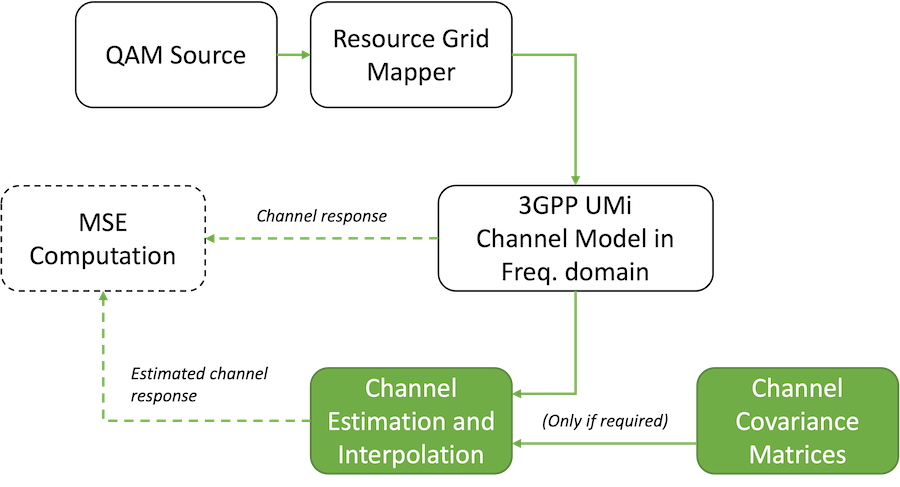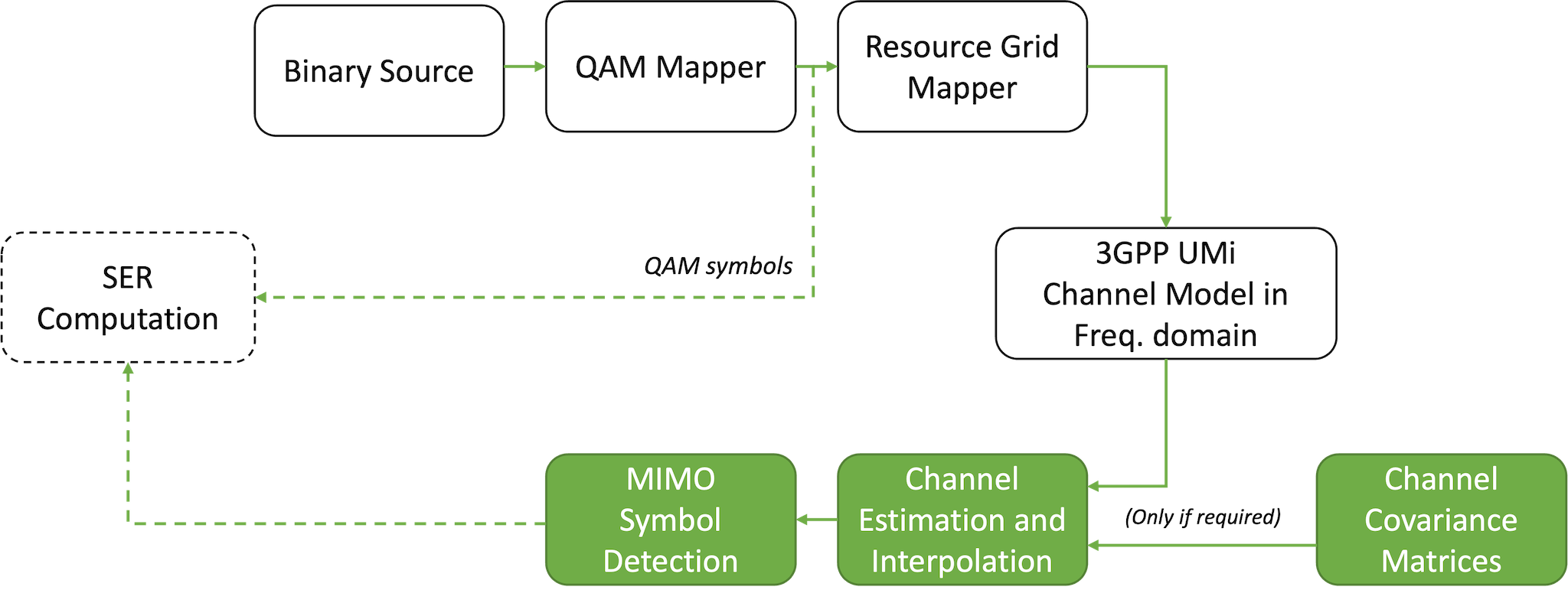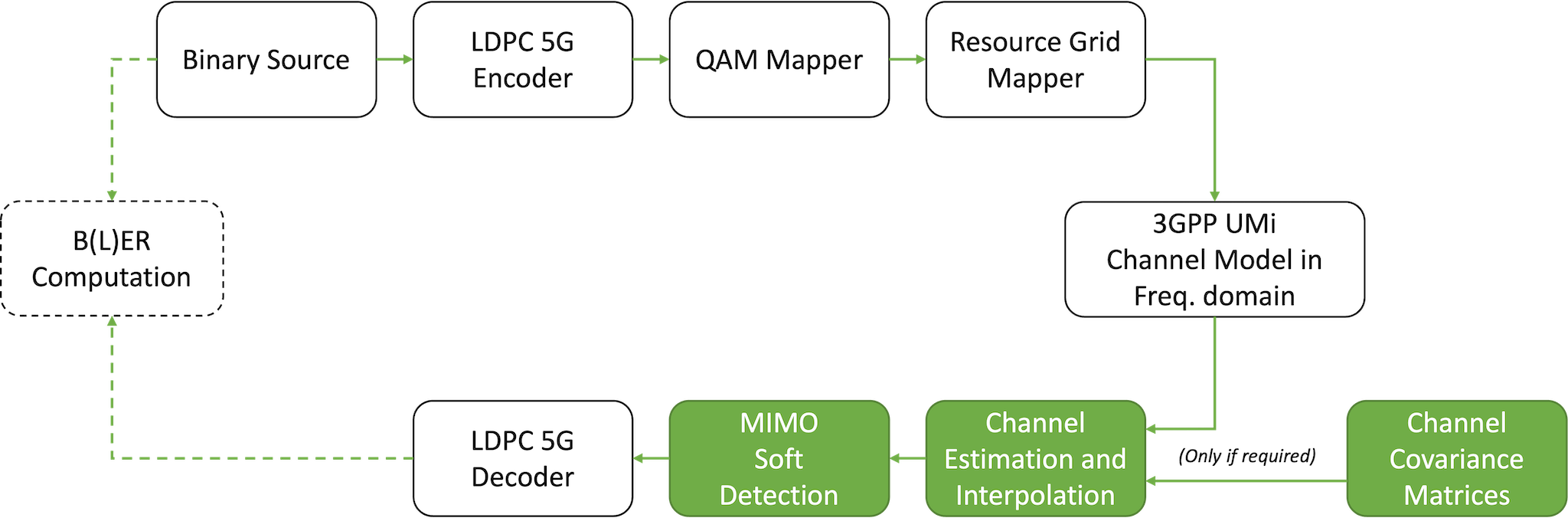Run in Google Colab View on GitHub Download notebook

# OFDM MIMO Channel Estimation and Detection

In this notebook, we will evaluate some of the OFDM channel estimation and MIMO detection algorithms available in Sionna.

We will start by evaluating the mean square error (MSE) preformance of various channel estimation and interpolation methods.

Then, we will compare some of the MIMO detection algorithms under both perfect and imperfect channel state information (CSI) in terms of uncoded symbol error rate (SER) and coded bit error rate (BER).

The developed end-to-end Keras models in this notebook are a great tool for benchmarking of MIMO receivers under realistic conditions. They can be easily extended to new channel estimation methods or MIMO detection algorithms.

For MSE evaluations, the block diagram of the system looks as follows:where the channel estimation module is highlighted as it is the focus of this evaluation. The channel covariance matrices are required for linear minimum mean square error (LMMSE) channel interpolation.

For uncoded SER evaluations, the block diagram of the system looks as follows:where the channel estimation and detection modules are highlighted as they are the focus of this evaluation.

Finally, for coded BER evaluations, the block diagram of the system looks as follows:## GPU Configuration and Imports

:

import os
gpu_num = 0 # Use "" to use the CPU
os.environ["CUDA_VISIBLE_DEVICES"] = f"{gpu_num}"
os.environ['TF_CPP_MIN_LOG_LEVEL'] = '3'
# Import Sionna
try:
import sionna
except ImportError as e:
# Install Sionna if package is not already installed
import os
os.system("pip install sionna")
import sionna

# Configure the notebook to use only a single GPU and allocate only as much memory as needed
# For more details, see https://www.tensorflow.org/guide/gpu
import tensorflow as tf
gpus = tf.config.list_physical_devices('GPU')
if gpus:
try:
tf.config.experimental.set_memory_growth(gpus, True)
except RuntimeError as e:
print(e)
# Avoid warnings from TensorFlow
tf.get_logger().setLevel('ERROR')

:

%matplotlib inline
import matplotlib.pyplot as plt
import numpy as np
import pickle

from tensorflow.keras import Model

from sionna.mimo import StreamManagement
from sionna.utils import QAMSource, compute_ser, BinarySource, sim_ber, ebnodb2no, QAMSource
from sionna.mapping import Mapper
from sionna.ofdm import ResourceGrid, ResourceGridMapper, LSChannelEstimator, LMMSEInterpolator, LinearDetector, KBestDetector, EPDetector, MMSEPICDetector
from sionna.channel import GenerateOFDMChannel, OFDMChannel, gen_single_sector_topology
from sionna.channel.tr38901 import UMi, Antenna, PanelArray
from sionna.fec.ldpc import LDPC5GEncoder
from sionna.fec.ldpc import LDPC5GDecoder


## Simulation parameters

The next cell defines the simulation parameters used throughout this notebook.

This includes the OFDM waveform parameters, antennas geometries and patterns, and the 3GPP UMi channel model.

:

NUM_OFDM_SYMBOLS = 14
FFT_SIZE = 12*4 # 4 PRBs
SUBCARRIER_SPACING = 30e3 # Hz
CARRIER_FREQUENCY = 3.5e9 # Hz
SPEED = 3. # m/s

# The user terminals (UTs) are equipped with a single antenna
# with vertial polarization.
UT_ANTENNA = Antenna(polarization='single',
polarization_type='V',
antenna_pattern='omni', # Omnidirectional antenna pattern
carrier_frequency=CARRIER_FREQUENCY)

# The base station is equipped with an antenna
# array of 8 cross-polarized antennas,
# resulting in a total of 16 antenna elements.
NUM_RX_ANT = 16
BS_ARRAY = PanelArray(num_rows_per_panel=4,
num_cols_per_panel=2,
polarization='dual',
polarization_type='cross',
antenna_pattern='38.901', # 3GPP 38.901 antenna pattern
carrier_frequency=CARRIER_FREQUENCY)

# 3GPP UMi channel model is considered
CHANNEL_MODEL = UMi(carrier_frequency=CARRIER_FREQUENCY,
o2i_model='low',
ut_array=UT_ANTENNA,
bs_array=BS_ARRAY,
enable_pathloss=False)


## Estimation of the channel time, frequency, and spatial covariance matrices

The linear minimum mean square (LMMSE) interpolation method requires knowledge of the time (i.e., across OFDM symbols), frequency (i.e., across sub-carriers), and spatial (i.e., across receive antennas) covariance matrices of the channel frequency response.

These are estimated in this section using Monte Carlo sampling.

We explain below how this is achieved for the frequency covariance matrix. The same approach is used for the time and spatial covariance matrices.

Let $$N$$ be the number of sub-carriers. The first step for estimating the frequency covariance matrix is to sample the channel model in order to build a set of frequency-domain channel realizations $$\left\{ \mathbf{h}_k \right\}, 1 \leq k \leq K$$, where $$K$$ is the number of samples and $$\mathbf{h}_k \in \mathbb{C}^{N}$$ are complex-valued samples of the channel frequency response.

The frequency covariance matrix $$\mathbf{R}^{(f)} \in \mathbb{C}^{N \times N}$$ is then estimated by

\begin{equation} \mathbf{R}^{(f)} \approx \frac{1}{K} \sum_{k = 1}^K \mathbf{h}_k \mathbf{h}_k^{\mathrm{H}} \end{equation}

where we assume that the frequency-domain channel response has zero mean.

The following cells implement this process for all three dimensions (frequency, time, and space).

The next cell defines a resource grid and an OFDM channel generator for sampling the channel in the frequency domain.

:

rg = ResourceGrid(num_ofdm_symbols=NUM_OFDM_SYMBOLS,
fft_size=FFT_SIZE,
subcarrier_spacing=SUBCARRIER_SPACING)
channel_sampler = GenerateOFDMChannel(CHANNEL_MODEL, rg)


Then, a function that samples the channel is defined. It randomly samples a network topology for every batch and for every batch example using the appropriate utility function.

:

def sample_channel(batch_size):
# Sample random topologies
topology = gen_single_sector_topology(batch_size, 1, 'umi', min_ut_velocity=SPEED, max_ut_velocity=SPEED)
CHANNEL_MODEL.set_topology(*topology)

# Sample channel frequency responses
# [batch size, 1, num_rx_ant, 1, 1, num_ofdm_symbols, fft_size]
h_freq = channel_sampler(batch_size)
# [batch size, num_rx_ant, num_ofdm_symbols, fft_size]
h_freq = h_freq[:,0,:,0,0]

return h_freq


We now define a function that estimates the frequency, time, and spatial covariance matrcies using Monte Carlo sampling.

:

@tf.function(jit_compile=True) # Use XLA for speed-up
def estimate_covariance_matrices(num_it, batch_size):
freq_cov_mat = tf.zeros([FFT_SIZE, FFT_SIZE], tf.complex64)
time_cov_mat = tf.zeros([NUM_OFDM_SYMBOLS, NUM_OFDM_SYMBOLS], tf.complex64)
space_cov_mat = tf.zeros([NUM_RX_ANT, NUM_RX_ANT], tf.complex64)
for _ in tf.range(num_it):
# [batch size, num_rx_ant, num_ofdm_symbols, fft_size]
h_samples = sample_channel(batch_size)

#################################
# Estimate frequency covariance
#################################
# [batch size, num_rx_ant, fft_size, num_ofdm_symbols]
h_samples_ = tf.transpose(h_samples, [0,1,3,2])
# [batch size, num_rx_ant, fft_size, fft_size]
# [fft_size, fft_size]
freq_cov_mat_ = tf.reduce_mean(freq_cov_mat_, axis=(0,1))
# [fft_size, fft_size]
freq_cov_mat += freq_cov_mat_

################################
# Estimate time covariance
################################
# [batch size, num_rx_ant, num_ofdm_symbols, fft_size]
# [num_ofdm_symbols, num_ofdm_symbols]
time_cov_mat_ = tf.reduce_mean(time_cov_mat_, axis=(0,1))
# [num_ofdm_symbols, num_ofdm_symbols]
time_cov_mat += time_cov_mat_

###############################
# Estimate spatial covariance
###############################
# [batch size, num_ofdm_symbols, num_rx_ant, fft_size]
h_samples_ = tf.transpose(h_samples, [0,2,1,3])
# [batch size, num_ofdm_symbols, num_rx_ant, num_rx_ant]
# [num_rx_ant, num_rx_ant]
space_cov_mat_ = tf.reduce_mean(space_cov_mat_, axis=(0,1))
# [num_rx_ant, num_rx_ant]
space_cov_mat += space_cov_mat_

freq_cov_mat /= tf.complex(tf.cast(NUM_OFDM_SYMBOLS*num_it, tf.float32), 0.0)
time_cov_mat /= tf.complex(tf.cast(FFT_SIZE*num_it, tf.float32), 0.0)
space_cov_mat /= tf.complex(tf.cast(FFT_SIZE*num_it, tf.float32), 0.0)

return freq_cov_mat, time_cov_mat, space_cov_mat


We then compute the estimates by executing the function defined in the previous cell.

The batch size and number of iterations determine the total number of samples, i.e.,

number of samples = batch_size x num_iterations


and hence control the tradeoff between the accuracy of the estimates and the time needed for their computation.

:

batch_size = 1000
num_iterations = 100

sionna.Config.xla_compat = True # Enable Sionna's support of XLA
FREQ_COV_MAT, TIME_COV_MAT, SPACE_COV_MAT = estimate_covariance_matrices(batch_size, num_iterations)
sionna.Config.xla_compat = False # Disable Sionna's support of XLA


Finally, the estimated matrices are saved (as numpy arrays) for future use.

:

# FREQ_COV_MAT : [fft_size, fft_size]
# TIME_COV_MAT : [num_ofdm_symbols, num_ofdm_symbols]
# SPACE_COV_MAT : [num_rx_ant, num_rx_ant]

np.save('freq_cov_mat', FREQ_COV_MAT.numpy())
np.save('time_cov_mat', TIME_COV_MAT.numpy())
np.save('space_cov_mat', SPACE_COV_MAT.numpy())


The next cell loads saved estimates of the time, frequency, and space covariance matrices.

:

FREQ_COV_MAT = np.load('freq_cov_mat.npy')


We then visualize the loaded matrices.

As one can see, the frequency correlation slowly decays with increasing spectral distance.

The time-correlation is much stronger as the mobility low. The covariance matrix is hence very badly conditioned with rank almost equal to one.

The spatial covariance matrix has a regular structure which is determined by the array geometry and polarization of its elements.

:

fig, ax = plt.subplots(3,2, figsize=(10,12))
fig.suptitle("Time and frequency channel covariance matrices")

ax[0,0].set_title("Freq. cov. Real")
im = ax[0,0].imshow(FREQ_COV_MAT.real, vmin=-0.3, vmax=1.8)
ax[0,1].set_title("Freq. cov. Imag")
im = ax[0,1].imshow(FREQ_COV_MAT.imag, vmin=-0.3, vmax=1.8)

ax[1,0].set_title("Time cov. Real")
im = ax[1,0].imshow(TIME_COV_MAT.real, vmin=-0.3, vmax=1.8)
ax[1,1].set_title("Time cov. Imag")
im = ax[1,1].imshow(TIME_COV_MAT.imag, vmin=-0.3, vmax=1.8)

ax[2,0].set_title("Space cov. Real")
im = ax[2,0].imshow(SPACE_COV_MAT.real, vmin=-0.3, vmax=1.8)
ax[2,1].set_title("Space cov. Imag")
im = ax[2,1].imshow(SPACE_COV_MAT.imag, vmin=-0.3, vmax=1.8)

cbar_ax = fig.add_axes([0.85, 0.15, 0.05, 0.7])
fig.colorbar(im, cax=cbar_ax);

:

<matplotlib.colorbar.Colorbar at 0x7effa863f0d0>## Comparison of OFDM estimators

This section focuses on comparing the available OFDM channel estimators in Sionna for the considered setup.

OFDM channel estimation consists of two steps:

1. Channel estimation at pilot-carrying resource elements using least-squares (LS).

2. Interpolation for data-carrying resource elements, for which three methods are available in Sionna:

• Nearest-neighbor, which uses the channel estimate of the nearest pilot

• Linear, with optional averaging over the OFDM symbols (time dimension) for low mobility scenarios

• LMMSE, which requires knowledge of the time and frequency covariance matrices

The LMMSE interpolator also features optional spatial smoothin, which requires the spatial covarance matrix. The API documentation explains in more detail how this interpolator operates.

### End-to-end model

In the next cell, we will create a Keras model which uses the interpolation method specified at initialization.

It computes the mean square error (MSE) for a specified batch size and signal-to-noise ratio (SNR) (in dB).

The following interpolation methods are available (set through the int_method parameter):

• "nn" : Nearest-neighbor interpolation

• "lin" : Linear interpolation

• "lmmse" : LMMSE interpolation

When LMMSE interpolation is used, it is required to specified the order in which interpolation and optional spatial smoothing is performed. This is achieved using the lmmse_order parameter. For example, setting this parameter to "f-t" leads to frequency interpolation being performed first followed by time interpolation, and no spatial smoothing. Setting it to "t-f-s" leads to time interpolation being performed first, followed by frequency interpolation, and finally spatial smoothing.

:

class MIMOOFDMLink(Model):

def __init__(self, int_method, lmmse_order=None, **kwargs):
super().__init__(kwargs)

assert int_method in ('nn', 'lin', 'lmmse')

# Configure the resource grid
rg = ResourceGrid(num_ofdm_symbols=NUM_OFDM_SYMBOLS,
fft_size=FFT_SIZE,
subcarrier_spacing=SUBCARRIER_SPACING,
num_tx=1,
pilot_pattern="kronecker",
pilot_ofdm_symbol_indices=[2,11])
self.rg = rg

# Stream management
# Only a sinlge UT is considered for channel estimation
sm = StreamManagement([], 1)

##################################
# Transmitter
##################################

self.qam_source = QAMSource(num_bits_per_symbol=2) # Modulation order does not impact the channel estimation. Set to QPSK
self.rg_mapper = ResourceGridMapper(rg)

##################################
# Channel
##################################

self.channel = OFDMChannel(CHANNEL_MODEL, rg, return_channel=True)

###################################
###################################

# Channel estimation
freq_cov_mat = tf.constant(FREQ_COV_MAT, tf.complex64)
time_cov_mat = tf.constant(TIME_COV_MAT, tf.complex64)
space_cov_mat = tf.constant(SPACE_COV_MAT, tf.complex64)
if int_method == 'nn':
self.channel_estimator = LSChannelEstimator(rg, interpolation_type='nn')
elif int_method == 'lin':
self.channel_estimator = LSChannelEstimator(rg, interpolation_type='lin')
elif int_method == 'lmmse':
lmmse_int_freq_first = LMMSEInterpolator(rg.pilot_pattern, time_cov_mat, freq_cov_mat, space_cov_mat, order=lmmse_order)
self.channel_estimator = LSChannelEstimator(rg, interpolator=lmmse_int_freq_first)

@tf.function
def call(self, batch_size, snr_db):

##################################
# Transmitter
##################################

x = self.qam_source([batch_size, 1, 1, self.rg.num_data_symbols])
x_rg = self.rg_mapper(x)

##################################
# Channel
##################################

no = tf.pow(10.0, -snr_db/10.0)
topology = gen_single_sector_topology(batch_size, 1, 'umi', min_ut_velocity=SPEED, max_ut_velocity=SPEED)
CHANNEL_MODEL.set_topology(*topology)
y_rg, h_freq = self.channel((x_rg, no))

###################################
# Channel estimation
###################################

h_hat,_ = self.channel_estimator((y_rg,no))

###################################
# MSE
###################################

mse = tf.reduce_mean(tf.square(tf.abs(h_freq-h_hat)))

return mse


The next cell defines a function for evaluating the mean square error (MSE) of a model over a range of SNRs (snr_dbs).

The batch_size and num_it parameters control the number of samples used to compute the MSE for each SNR value.

:

def evaluate_mse(model, snr_dbs, batch_size, num_it):

# Casting model inputs to TensorFlow types to avoid
# re-building of the graph
snr_dbs = tf.cast(snr_dbs, tf.float32)
batch_size = tf.cast(batch_size, tf.int32)

mses = []
for snr_db in snr_dbs:

mse_ = 0.0
for _ in range(num_it):
mse_ += model(batch_size, snr_db).numpy()
# Averaging over the number of iterations
mse_ /= float(num_it)
mses.append(mse_)

return mses


The next cell defines the evaluation parameters.

:

# Range of SNR (in dB)
SNR_DBs = np.linspace(-10.0, 20.0, 20)

# Number of iterations and batch size.
# These parameters control the number of samples used to compute each SNR value.
# The higher the number of samples is, the more accurate the MSE estimation is, at
# the cost of longer compute time.
BATCH_SIZE = 512
NUM_IT = 10

# Interpolation/filtering order for the LMMSE interpolator.
# All valid configurations are listed.
# Some are commented to speed-up simulations.
# Uncomment configurations to evaluate them!
ORDERS = ['s-t-f', # Space - time - frequency
#'s-f-t', # Space - frequency - time
#'t-s-f', # Time - space - frequency
't-f-s', # Time - frequency - space
#'f-t-s', # Frequency - time - space
#'f-s-t', # Frequency - space- time
#'f-t',   # Frequency - time (no spatial smoothing)
't-f'   # Time - frequency (no spatial smoothing)
]


The next cell evaluates the nearest-neighbor, linear, and LMMSE interpolator. For the LMMSE interpolator, we loop through the configuration listed in ORDERS.

:

MSES = {}

# Nearest-neighbor interpolation
MSES['nn'] = evaluate_mse(e2e, SNR_DBs, BATCH_SIZE, NUM_IT)

# Linear interpolation
MSES['lin'] = evaluate_mse(e2e, SNR_DBs, BATCH_SIZE, NUM_IT)

# LMMSE
for order in ORDERS:
MSES[f"lmmse: {order}"] = evaluate_mse(e2e, SNR_DBs, BATCH_SIZE, NUM_IT)


WARNING:tensorflow:From /home/faycal/.local/lib/python3.8/site-packages/tensorflow/python/util/dispatch.py:1176: calling gather (from tensorflow.python.ops.array_ops) with validate_indices is deprecated and will be removed in a future version.
Instructions for updating:
The validate_indices argument has no effect. Indices are always validated on CPU and never validated on GPU.


Finally, we plot the MSE.

:

plt.figure(figsize=(8,6))

for est_label in MSES:
plt.semilogy(SNR_DBs, MSES[est_label], label=est_label)

plt.xlabel(r"SNR (dB)")
plt.ylabel("MSE")
plt.legend()
plt.grid(True)Unsurprisingly, the LMMSE interpolator leads to more accurate estimates compared to the two other methods, as it leverages knowledge of the the channel statistics. Moreover, the order in which the LMMSE interpolation steps are performed strongly impacts the accuracy of the estimator. This is because the LMMSE interpolation operates in one dimension at a time which is not equivalent to full-blown LMMSE estimation across all dimensions at one.

Also note that the order that leads to the best accuracy depends on the channel statistics. As a rule of thumb, it might be good to start with the dimension that is most strongly correlated (i.e., time in our example).

## Comparison of MIMO detectors

An OFDM MIMO receiver consists of two stages: OFDM channel estimation and MIMO detection.

While the previous section focused on OFDM channel estimation, this section focuses now on MIMO detection.

The following MIMO detection algorithms, all available out-of-the-box in Sionna, are considered:

Both perfect and imperfect channel state information is considered in the simulations. LS estimation combined with LMMSE interpolation is used, with time-frequency-space smoothing (in this order, i.e., order='t-f-s').

### End-to-end model

A Keras model is created in the next cell, which uses the detection method specified at initialization.

It computes either the coded bit error rate (BER) or the uncoded symbol error rate (SER), for a specified batch size, $$E_b/N_0$$ (in dB), and QAM modulation with a specified modulation order. When computing the BER, a 5G LDPC code is used with the specified coderate.

The following MIMO detection methods are considered (set through the det_param parameter):

• "lmmse" : No parameter needed

• "k-best" : List size k, defaults to 64

• "ep" : Number of iterations l, defaults to 10

• "mmse-pic" : Number of self-iterations num_it, defaults to 4

The det_param parameter corresponds to either k, l, or num_it, for K-Best, EP, or MMSE-PIC, respectively. If set to None, a default value is used according to the selected detector.

The perf_csi parameter controls whether perfect CSI is assumed or not. If set to False, then LS combined with LMMSE interpolation is used to estimate the channel.

You can easily add your own MIMO detector and channel estimator to this model for a fair and realistic benchmark.

:

class MIMOOFDMLink(Model):

def __init__(self, output, det_method, perf_csi, num_tx, num_bits_per_symbol, det_param=None, coderate=0.5, **kwargs):
super().__init__(kwargs)

assert det_method in ('lmmse', 'k-best', 'ep', 'mmse-pic'), "Unknown detection method"

self._output = output
self.num_tx = num_tx
self.num_bits_per_symbol = num_bits_per_symbol
self.coderate = coderate
self.det_method = det_method
self.perf_csi = perf_csi

# Configure the resource grid
rg = ResourceGrid(num_ofdm_symbols=NUM_OFDM_SYMBOLS,
fft_size=FFT_SIZE,
subcarrier_spacing=SUBCARRIER_SPACING,
num_tx=num_tx,
pilot_pattern="kronecker",
pilot_ofdm_symbol_indices=[2,11])
self.rg = rg

# Stream management
sm = StreamManagement(np.ones([1,num_tx], int), 1)

# Codeword length and number of information bits per codeword
n = int(rg.num_data_symbols*num_bits_per_symbol)
k = int(coderate*n)
self.n = n
self.k = k

# If output is symbol, then no FEC is used and hard decision are output
hard_out = (output == "symbol")
coded = (output == "bit")
self.hard_out = hard_out
self.coded = coded

##################################
# Transmitter
##################################

self.binary_source = BinarySource()
self.mapper = Mapper(constellation_type="qam", num_bits_per_symbol=num_bits_per_symbol, return_indices=True)
self.rg_mapper = ResourceGridMapper(rg)
if coded:
self.encoder = LDPC5GEncoder(k, n, num_bits_per_symbol=num_bits_per_symbol)

##################################
# Channel
##################################

self.channel = OFDMChannel(CHANNEL_MODEL, rg, return_channel=True)

###################################
###################################

# Channel estimation
if not self.perf_csi:
freq_cov_mat = tf.constant(FREQ_COV_MAT, tf.complex64)
time_cov_mat = tf.constant(TIME_COV_MAT, tf.complex64)
space_cov_mat = tf.constant(SPACE_COV_MAT, tf.complex64)
lmmse_int_time_first = LMMSEInterpolator(rg.pilot_pattern, time_cov_mat, freq_cov_mat, space_cov_mat, order='t-f-s')
self.channel_estimator = LSChannelEstimator(rg, interpolator=lmmse_int_time_first)

# Detection
if det_method == "lmmse":
self.detector = LinearDetector("lmmse", output, "app", rg, sm, constellation_type="qam", num_bits_per_symbol=num_bits_per_symbol, hard_out=hard_out)
elif det_method == 'k-best':
if det_param is None:
k = 64
else:
k = det_param
self.detector = KBestDetector(output, num_tx, k, rg, sm, constellation_type="qam", num_bits_per_symbol=num_bits_per_symbol, hard_out=hard_out)
elif det_method == "ep":
if det_param is None:
l = 10
else:
l = det_param
self.detector = EPDetector(output, rg, sm, num_bits_per_symbol, l=l, hard_out=hard_out)
elif det_method == 'mmse-pic':
if det_param is None:
l = 4
else:
l = det_param
self.detector = MMSEPICDetector(output, rg, sm, 'app', num_iter=l, constellation_type="qam", num_bits_per_symbol=num_bits_per_symbol, hard_out=hard_out)

if coded:
self.decoder = LDPC5GDecoder(self.encoder, hard_out=False)

@tf.function
def call(self, batch_size, ebno_db):

##################################
# Transmitter
##################################

if self.coded:
b =